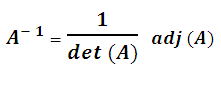# C and C++ Program to Find Inverse of a Matrix

Here you will get C and C++ program to find inverse of a matrix.

We can obtain matrix inverse by following method.

• First calculate deteminant of matrix.
• Then calculate adjoint of given matrix. Adjoint can be obtained by taking transpose of cofactor matrix of given square matrix.
• Finally multiply 1/deteminant by adjoint to get inverse.

The formula to find inverse of matrix is given below.You can watch below video to learn how inverse is calculated.

In below program I have calculated the inverse of 3×3 matrix.

## C++ Program to Find Inverse of a Matrix

Output### 17 thoughts on “C and C++ Program to Find Inverse of a Matrix”

1.Someone once need help

thank you very much for your example, now i can go to bed easily =D . Really help my task to do inverse matrices from read files

2.why you use %3 finding determinant

1.Matrix size 3

2.%3 keeps the for loop from trying to access out of bound array

3.Write a program in C++ to find the minor of every elements of a square matrix of any order.
The order of the square matrix should be entered by the user.

4.what is the function of the mode in the code

5.Thank u so much dude… u’ve helped us..

6.I did not understand this can you explain me clear ‘
cout<<((mat[(j+1)%3][(i+1)%3] * mat[(j+2)%3][(i+2)%3]) – (mat[(j+1)%3][(i+2)%3] * mat[(j+2)%3][(i+1)%3]))/ determinant<<"\t";

what is J+1, i+1 and so on?

7.Thank you very much to upload this program

8.Bro i want the explanation about the full program if u have any related videos plz send to my mail

9.What would be the changes to calculate 4*4 matrix or further ?

10.i don’t understand (j+1)%3 and (i+1)%3

11.So wise! I was trying to implement the same thing by calculating each element manually and that could take forever.. Thank you so much!

12.Can you please give me the pseudo code for this?

13.May for such a simple and awesome code I’ve wasted hours in searching from sites to sites.
Live long sir!

14.Easy to Understand Code It is just Like what the teacher taught me during jee preparation’;
I want to solve it using the rank method

15.lol im actually confused on how the c++ program is outputting the inverse array in decimal form when the array itself is an int datatype